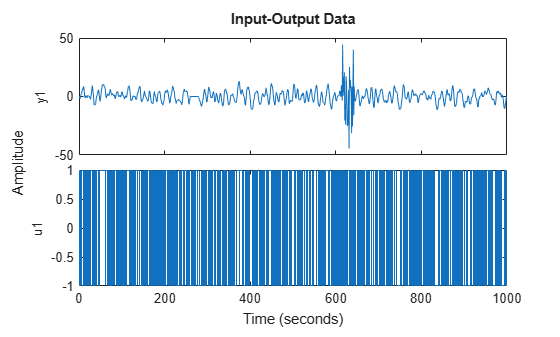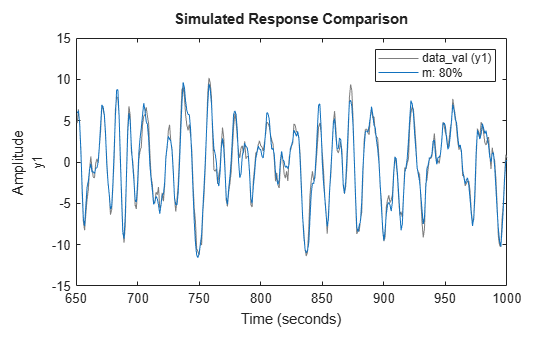Main Content

# Extract and Model Specific Data Segments

This example shows how to create a multi-experiment, time-domain data set by merging only the accurate data segments and ignoring the rest.

Load and plot the data.

```load iddemo8; plot(dat);```The data has poor or no measurements from samples 251 to 280 and 601 to 650. You cannot simply concatenate the good data segments because the transients at the connection points compromise the model. Instead, you must create a multiexperiment `iddata` object, where each experiment corresponds to a good segment of data.

Create multiexperiment data set by merging data segments.

`datam = merge(dat(1:250),dat(281:600),dat(651:1000));`

Estimate a state-space model using the multiexperiment data set using experiments 1 and 2.

```data_est = getexp(datam,[1,2]); m = ssest(data_est,2);```

Validate the model by comparing its output to the output data of experiment 3.

```data_val = getexp(datam,3); compare(data_val,m)```## Support평가판 신청